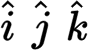## Friday, October 30, 2020

### Scalars and Vectors

Please note that whenever I mentioned i j and k in a sentence I am referring to the special unit vectors:.

A scalar is a quantity that possesses only magnitude. Some example of scalar quantities includes mass, length, time, distance, speed, and density.

A vector is a quantity that possesses both magnitude and direction. Examples of vector quantities are displacement, velocity, acceleration, and force. A vector quantity can be represented by an arrow drawn to scale. The length of the arrow is proportional to the magnitude of the vector quantity. The direction of the arrow represents the direction of the vector quantity.

The Components of a Vector

Before we define the components of a vector, we first must introduce the elementary relationships between trigonometric functions. The trigonometric functions are defined in relation to a right angle. For the right triangle shown below by definition.We often use these in the forms

A component of a vector is its effective value in a given direction. For example, the x-component of displacement is the displacement parallel to the x-axis caused by the given displacement. A vector in three dimensions may be considered as the resultant of its component vectors resolved along with any three mutually perpendicular directions. Similarly, a vector in two dimensions may be resolved into two component vectors acting along any two mutually perpendicular directions. The picture below shows the vector R and its x and y vector components, Rx, Ry, which have magnitudesUnit Vectors

Unit vectors have a magnitude of one and are represented by a boldface symbol topped with a caret. The special unit vector (see below) are assigned to the x-, y-, and x-axes, respectively. A vector 3 i represents a three-unit vector in the +x direction, while -5 k represents a five-unit vector in the -z direction.A vector R that has scalar x-, y-, and x-components Rx, Ry, and Rz, respectively, can be written as

When an object moves from one point in space to another, the displacement is the vector from the initial location to the final location. It is independent of the actual distance traveled.